## 6.2.4 Secondary Electron Deflection

Expected secondary electron yields of a conversion surface are in the range of 0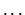1 electrons per incident particle in the energy range under investigation (101000eV per atom). Since these electrons might interfere with the measurement of negative ions a deflection magnet was built which would cause a magnetic field perpendicular to the incoming neutral particle beam and parallel to the surface. It was decided that the gyro radius of a low energy electron (E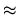2eV) next to the surface should be of the order of 1mm whereas oxygen atoms with an energy of200eV should not be deflected at all. The necessary strength of the field is calculated by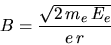(6.4)

where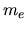denotes the electron mass,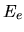the energy of the electron,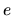the elementary charge,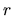the gyro radius, and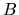the magnetic flux density.

The necessary flux densitycomputes to 3.3 mT. At this flux density protons and oxygen atoms are only marginally deflected. For the dimensioning of the magnet a simple approach giving a field of the right order of magnitude was chosen. For an electro-magnet with an iron core, and a small air gap with a moderate and approximately homogeneous field in the air gap the magnetic resistivity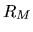is defined by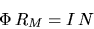(6.5)

where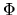is the total magnetic flux,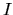the current in the coil, and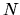the number of turns on the coil. The magnetic resistivity may be written as the sum of the resistivity of the iron core and the resistivity of the air gap: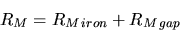(6.6)

for a homogeneous material and a homogeneous field the magnetic resistivity is calculated as follows: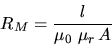(6.7)

where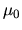is the permeability of vacuum,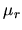the relative permeability of the material,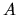the cross section of the iron core or the effective cross section of the air gap, and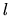the length of the medium. For the used type of steel St37is approximately 1000 compared to a value of 1 for vacuum (in the gap). This means that the contribution of the iron core to the magnetic resistivity can be neglected compared to the resistivity of the vacuum gap and Equations 6.5 and 6.6 simplify to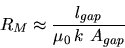(6.8)

where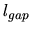denotes the width of the gap,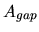the effective cross section of the gap, and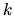a constant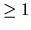. The main source of error in the calculation is that for a flattened horse shoe magnet with a wide gap the field in the gap is not homogeneous. This problem is addressed by the constant. For the geometry used for this magnet I used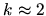. Combining Equation 6.5 and 6.8, and using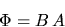(6.9)

the number of turns for the coil are calculated by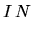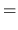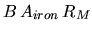(6.10)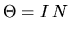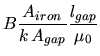(6.11)

With the mechanical dimensions of the magnet a magnetomotive force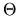= 67A was calculated. A copper wire with 0.3 mm diameter was used for the coil yielding four layers of wire within the available space and a total of 300 turns. The total resistance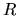is calculated by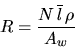(6.12)

withthe number of turns,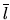the average length of one winding,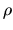the specific resistance, and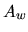the cross section of the wire. With the values used the resistance of the coil is 5.4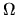.

DC power for the magnet was provided using a circuit shown in Figure 6.4.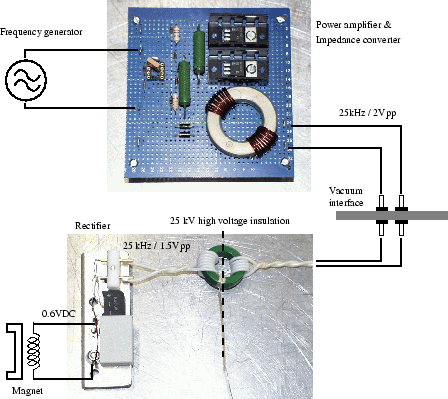A 25 kHz sine signal from a external waveform generator was fed into a balanced class A push-pull power amplifier realized using two bipolar transistors to lower the source impedance from 50to a few Ohm. This low impedance was needed to drive the high voltage insulation transformer inside the chamber. This transformer was built using a ferrite ring suitable for the 25 kHz. The primary and secondary coils were made using high voltage cables providing the 25 kV insulation needed. As a drawback only a few turns could be put on the ferrite ring because of the limited space available and the bulky cables. This was compensated by the rather high operating frequency of 25 kHz. On the high voltage side of the transformer a simple rectifier circuit converted the AC back to DC used to power the magnet.

A complete shielding around rectifier, capacitor, and coil of the magnet should minimize the danger of discharges to the high voltage side of the electronics (Figure 6.5).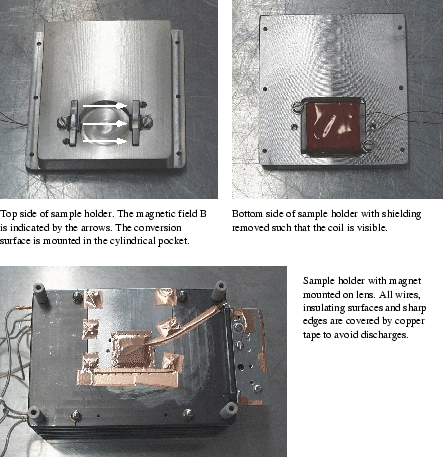Unfortunately during the measurements discharges occurred destroying the diodes in the rectifier once. After replacement no influence of the magnet on the measurements could be found but it was later discovered that a discharge had also short-circuited the coil of the magnet towards ground rendering the magnet useless. No replacement was installed during the measurement session because of the high amount of work needed to make a new coil. Fortunately the background was low enough to make measurements without the magnet.

March 2001 - Martin Wieser, Physikalisches Institut, University of Berne, Switzerland